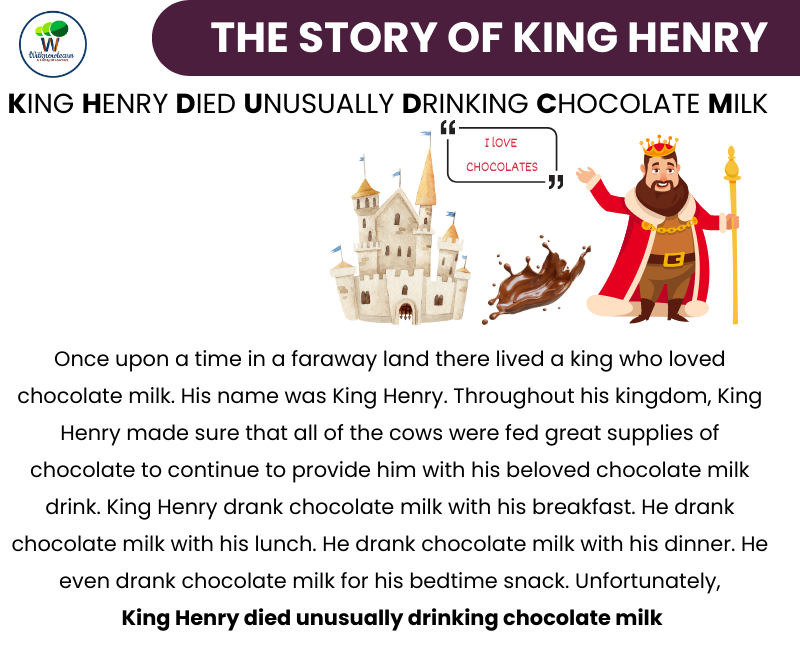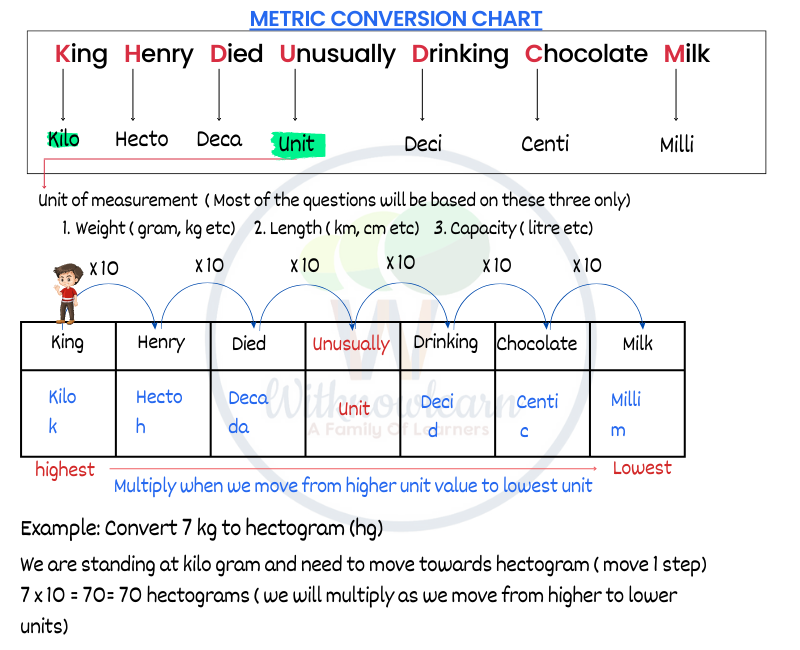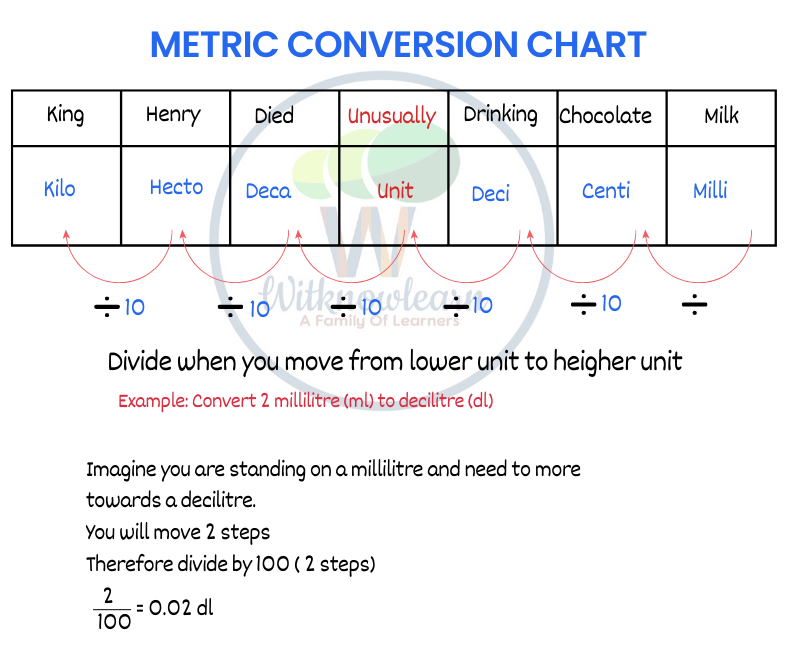# A Printable Worksheet on Conversion of Measurement – For Adults and Kids!Learn how to convert measurements with ease using this helpful worksheet! Designed for both adults and kids, it provides step-by-step instructions and explanations to help you easily understand the process of conversion.Understand the System of Measurement.

Understanding how the system of measurement works is essential if you want to accurately picture how much a unit is in another type of measurement. Before you attempt to convert measurements, it’s important to learn the system of measurement your calculations will use. One such example includes understanding that one foot is equal to 12 inches, or that 1 kilometer is equal to 0.62 miles. Once you have a grasp on the unit, you can move on to finding and calculating conversions.

Learn How to Convert Units with a Table.

When you think of converting units, a table is one of the best ways to do it. A conversion table helps condense multiple measurements into one easy-to-read chart. Most tables are organized in columns and feature a single unit on the left column, followed by its equivalent measurement in other types of units in subsequent columns. By understanding how the various measurements compare to each other, you can accurately convert between different units with ease.

Practice Converting Different Types of Measurements.

To get comfortable with converting between different types of measurements, you need to practice. On this worksheet, you can test your knowledge by converting linear distances and temperatures. Focus on solutions that require the conversion of the given value from bigger units to smaller ones. Examine how different numbers of smaller values can equal a single larger one and apply the knowledge when making conversions.

conversion of units plays a crucial role in various fields, including physics, mathematics, and everyday life. This indispensable tool helps us seamlessly transition between different systems of measurement. A table for conversion of units or a conversion of units chart simplifies the process by presenting the required information in an organized and easy-to-use format. These conversion of units tables can be found in a variety of formats, such as PDFs, making them readily accessible for anyone in need.

One common application of conversion of units is in length measurements. The conversion of units in length may involve converting between metric and imperial units, such as kilometers to miles or centimeters to inches. A conversion of units measurement table provides the necessary conversion factors to ensure accuracy and ease in calculations. Similarly, the conversion of units area and conversion of units length tables offer quick reference points for converting between square meters and square feet or meters and feet, respectively.

Conversion of units is not limited to length measurements. It also encompasses other domains, like the conversion of units of volume and weight. Conversion of units volume and conversion units of volume tables enable us to switch between liters and gallons or cubic meters and cubic feet with ease. Meanwhile, conversion of units weight charts assist in converting between mass measurements, such as grams to kilograms or pounds to ounces.

The realm of physics often requires the conversion of units, as well. In fact, conversion of units physics and conversion of units in physics are essential for understanding various concepts and solving complex problems. To help students, teachers often provide worksheets on conversion of units and conversion of units worksheets, which contain questions on conversion of units that allow learners to practice and hone their skills.

Learning how to conversion of units is a critical skill for students of all ages, starting as early as class 3 or class 4. By class 5 and class 6, students should be familiar with measurement and conversion of units, making use of conversion of units tricks and rules to simplify their work. As they progress, pupils in class 7 and above will encounter more advanced applications of conversion of units, such as in the context of physics or other scientific disciplines.

Conversion of units can be taught and learned in various languages, including Hindi, which makes the process accessible to a wider audience. Furthermore, students can employ a variety of learning objectives for conversion of units, such as using a worksheet for conversion of units or participating in an interactive conversion of units activity. Understanding how to solve conversion of units problems and how to calculate conversion units is integral to mastering this skill.

To assess one's understanding and proficiency in conversion of units, quizzes and questions on conversion of units can be used, often featuring conversion of units questions and answers. These resources help learners to further develop their conversion unit of measurement skills and solidify their understanding of conversion of units of measurement.

In conclusion, the conversion of units is a vital skill with applications in numerous fields, from everyday life to advanced scientific research. Resources such as conversion of units charts, tables, worksheets, and quizzes facilitate the learning process and make it more engaging. By mastering the art of converting units, individuals can overcome challenges associated with different measurement systems and confidently solve a wide range of problems.

FAQs

1. What is the purpose of conversion of units?

Conversion of units is the process of changing one unit of measurement to another, typically for purposes of comparison or calculation. It is essential for various fields, including mathematics, physics, and everyday life, to ensure accurate and consistent measurements.

1. What are the most common units of measurement systems?

The most common unit measurement systems are the Metric System (SI) and the Imperial System (US Customary). The Metric System uses units such as meters, grams, and liters, while the Imperial System uses units like feet, pounds, and gallons.

1. How do I convert between metric and imperial units?

To convert between metric and imperial units, you'll need to use a conversion factor, which is a multiplier used to change one unit to another. For example, to convert inches to centimeters, you would multiply the number of inches by 2.54, the conversion factor.

1. How do I use a conversion of units table or chart?

A conversion of units table or chart lists common conversion factors for various units of measurement. To use the table or chart, locate the unit you wish to convert from and the unit you want to convert to, then multiply your original measurement by the conversion factor provided.

1. Are there any shortcuts or tricks for conversion of units?

For certain conversions, especially within the metric system, there are shortcuts and tricks. For instance, converting between meters and kilometers can be done by simply moving the decimal point three places (1 kilometer = 1,000 meters). It is essential to understand the base units and their multiples to effectively use these shortcuts.

1. How can I practice conversion of units?

Worksheets, quizzes, and online resources offer ample opportunities to practice conversion of units. Many educational websites provide problems and exercises to help you build your skills and improve your understanding of the conversion process.

1. Why is conversion of units important in physics?

In physics, accurate and consistent measurements are crucial for understanding various concepts and solving problems. Conversion of units helps to ensure that all measurements are expressed in the same system, making calculations and comparisons more straightforward and reliable.

1. Are there any tools or apps for conversion of units?

Yes, there are many tools and apps available for conversion of units. These digital resources allow users to quickly convert between different units of measurement with ease. Some popular options include online converters, mobile apps, and software programs.

1. How can I teach conversion of units to younger students?

To teach conversion of units to younger students, start with basic concepts and use engaging, hands-on activities. Focus on common conversions they may encounter in everyday life, such as length or weight, and provide clear examples to illustrate the process.

1. Can I find conversion of units resources in other languages?

Yes, conversion of units resources can be found in various languages, including Hindi. This accessibility helps students from diverse linguistic backgrounds learn this essential skill, regardless of their native language.

Extra questions on conversion of units

Problem 1: Length Convert 5 kilometers to meters.

Solution: 1 kilometer = 1,000 meters 5 kilometers = 5 x 1,000 meters = 5,000 meters

Answer: 5 kilometers = 5,000 meters

Problem 2: Length Convert 32 centimeters to meters.

Solution: 1 meter = 100 centimeters 32 centimeters = 32 ÷ 100 meters = 0.32 meters

Answer: 32 centimeters = 0.32 meters

Problem 3: Weight Convert 7,500 grams to kilograms.

Solution: 1 kilogram = 1,000 grams 7,500 grams = 7,500 ÷ 1,000 kilograms = 7.5 kilograms

Answer: 7,500 grams = 7.5 kilograms

Problem 4: Weight Convert 15 pounds to ounces.

Solution: 1 pound = 16 ounces 15 pounds = 15 x 16 ounces = 240 ounces

Answer: 15 pounds = 240 ounces

Problem 5: Volume Convert 1,250 milliliters to liters.

Solution: 1 liter = 1,000 milliliters 1,250 milliliters = 1,250 ÷ 1,000 liters = 1.25 liters

Answer: 1,250 milliliters = 1.25 liters

Problem 6: Volume Convert 4 gallons to quarts.

Solution: 1 gallon = 4 quarts 4 gallons = 4 x 4 quarts = 16 quarts

Answer: 4 gallons = 16 quarts

Try these questions

Problem 1: Length Convert 400 centimeters to meters.

Problem 2: Length Convert 7 meters to centimeters.

Problem 3: Weight Convert 1,500 grams to kilograms.

Problem 4: Weight Convert 4 kilograms to grams.

Problem 5: Volume Convert 3,500 milliliters to liters.

Problem 6: Volume Convert 5 liters to milliliters.

• Tags :
• Conversion of units table